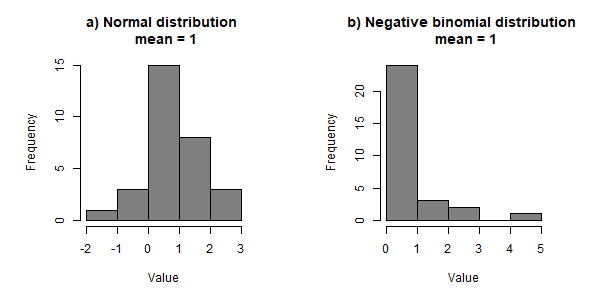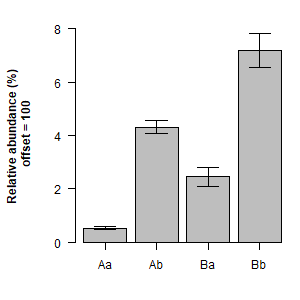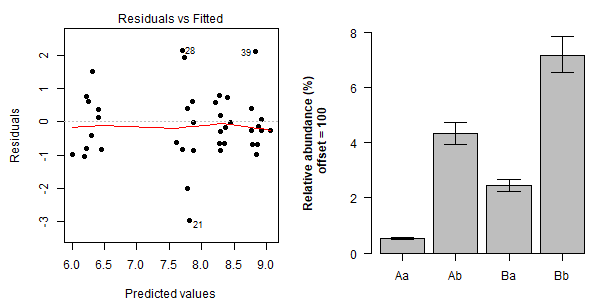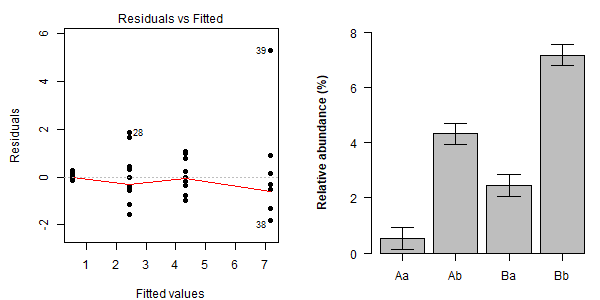# Offsets in GLMs

In many sequencing projects, we often have to deal with samples that have uneven sequencing depths. A popular approach was to rarefy the data such that the sequencing depths among each sample are the same, however it has been shown that this is less than optimal and modern statistical methods can use the full data sets we obtain (McMurdie and Holmes, 2014). McMurdie and Holmes (2014) suggest a number of R packages with these modern methods and built-in functions to do the analyses better (DESeq2 or EdgeR, but fail to mention MVAbund), but as someone who is quite familiar with these methods, I found the statistics were not adequately explained for a first time data analyser.

Let’s have a look at these modern methods, and how we can analyse our sequence counts with them.

## The suggestions

Various papers now suggest we used generalised linear models (GLMs) for our count data. Next we should use the total sequence counts per sample as an offset in our GLMs. What I further suggest is using the GLMs with an offset to obtain the expected count per 100, which without too far a stretch of the imagination, represents the relative abundance (%), and this is most intuitive to most researchers.

## Generalised linear models (GLMs)

You are probably familiar with t-tests and ANOVA, which are forms of a normal linear model and assume your data is normally distributed. The normal distribution is a continuous probability distribution, and works well in most cases, however it assumes you data is “continuous” which is unlike the “discrete” count data we obtain. Further, when our data approaches values near zero, the normal distribution will assume that our data can go below zero (figure 1a), and we know this cannot be true.

There are other mathematically derived distributions, and one important one is the negative binomial (NB) distribution. This is a discrete probability distribution, and in most cases, count data we obtain through sequencing is NB distributed. When our data approaches values near zero, the NB distribution will assume that our data be more likely zero (figure 1b), rather than cross into below zero values. The use the NB distribution we use generalised linear models. Fortunately, what we see in the outputs of generalised linear models is just about the same as with the normal linear model.Figure 1. Simulated data from a) the normal and b) negative binomial (NB) distributions with a mean of 1. The SD for the normal distribution was 1 and the dispersion of the NB distribution was 5.

### Offsets

One important feature of the NB model is the natural extension of modelling the rate of the counts. This is achieved using an offset term. Thus we can model the number of counts of a variable given the total number from which these counts were sampled. This is perfectly deals with our situation! We can now avoid rarefying the data, and model our counts given the total number of sequences from each sample from the full un-rarefied.

## An example

Here I have simulated some data (be it an OTU, gene, transcript or feature) that we can use to observe how the offset works in an NB glm. We will also se how a normal LM does.

### Simulation

# Set seed for reproducible randomisation
set.seed(1010)
# Create a vector of 40 random total sequence counts with mu = 100k and sd = 10k
TotalsVariation = round(rnorm(10 * 4, 100000, 10000))
# Set the mean rate for 4 groups
MeanRates = c(0.005, 0.05, 0.02, 0.07)
set.seed(1010)
# Create 10 NB random samples from the 4 groups, given the random total sequences and a dispersion of 10
Ys = rnegbin(10 * 4, rep(MeanRates*TotalsVariation, each = 10), 10)
# Create a factor vector
Groups = factor(rep(c("Aa", "Ab", "Ba", "Bb"), each = 10))
# Add all the data to a dataframe
Offset.dat = data.frame(Count = Ys, Groups = Groups, Totals = TotalsVariation))  

offset.dat is a dataframe containing a set of simulated counts (Counts) from a set of totals (Totals) among four groups (Groups). We can look at the data in term of relative abundance by calculating the means and SEs by hand and then plotting (Figure 2).

offset.dat$RelativeAbundance = offset.dat$Count / offset.dat$Totals * 100 Means = tapply(offset.dat$RelativeAbundance, offset.dat$Groups, mean) SE = tapply(offset.dat$RelativeAbundance, offset.dat$Groups, sd) / sqrt(10) library(gplots) barplot2(Means, plot.ci = T, ci.l = Means - SE, ci.u = Means + SE, las = 1, axis.lty = 1, ylab = "Relative abundance (%) \n offset = 100", font.lab = 2, ylim = c(0,8))Figure 2. Relative abundance of the simulated data. One thing we notice is that the SE increases with the mean. The smallest mean (Aa) also has the smallest mean, while the largest mean and SE (Bb). This is tends to happen with count data, which is expected in the NB distribution but not the normal distribution. ### NB GLM We can then fit a NB GLM using an offset. Note that the offset has to be on a log scale, as the NB GLM works on a log scale (but you do not need to transform your data) fit.nb = glm.nb(Count ~ Groups + offset(log(Totals)), data = offset.dat) I’m going to leave model summaries and ANOVAs for another day, and only look at the residual vs. fits and then jump straight to the model predictions - or in other words, the means and SE of the data. What the residuals plot (Figure 3a) tells us is how well the data fit the model we made. What we want to see is no pattern in the points (a first time user will surely ask ‘what?’, but take it for granted now), and that is what we see - our predicted values (what our model will tell us) fit nicely with the data, and the residuals (the difference between the model vs real data) are even across all predicted values i.e. no pattern. We can then obtain our means and SE, which at this stage are on a log scale. To do this in R, we need to have a dataframe with the model predictors/covariate for which to obtain the data we want. We make a column of the dataframe called ‘Groups’ (as in the model above) with the levels of factors in Groups. Next we need a column for the offset value. If we use 100, then we are asking for the estimate count for each group given 100 total counts. Sounds very much like relative abundance to me! We then run predict, which gives us a list with one object a matrix of means, the another a matrix of SEs. Mean.dat = data.frame(Groups = levels(offset.dat$Groups), Totals = 100)
Preds.list = predict(fit.nb, Mean.dat, se.fit = T) 

We can easily plot this data (Figure 3b), noting that we should take the exponents to go back to the response scale (the counts). The upper and lower SE bound are made by adding or subtracting the SE from the mean and then exponentiating the result. The figure looks very much like the relative abundance figure that we made via hand calculations. Nice!

Plot.dat = rbind(Mean = exp(Preds.list$fit), Upper = exp(Preds.list$fit + Preds.list$se.fit), Lower = exp(Preds.list$fit - Preds.list$se.fit)) colnames(Plot.dat) = levels(offset.dat$Groups)
barplot2(Plot.dat["Mean",], plot.ci = T, ci.l = Plot.dat["Lower",], ci.u = Plot.dat["Upper",],
las = 1, axis.lty = 1, ylab = "Relative abundance (%) \n offset = 100", font.lab = 2,
ylim = c(0,8))Figure 3. a) residual plot of NB model and b) predictions (mean and SE) of the given model using an offset = 100.

### A normal linear model

Lets see the output from doing a normal linear model (code not shown). Notice that we see a pattern in the residuals (Figure 4a), as the predicted values get larger so do the residuals. This is a bad thing, such that the model does not fit the data, or vice versa. Next, plotting the means and SEs show that the means seem ok but the SEs do not.Figure 4. a) residual plot of normal linear model and b) predictions (mean and SE) of the given model

## Conclusion

I would have to say that I am pretty convinced that NB GLMs should be used for count data from sequencing projects. The option to use an offset means we no longer need to rarefy and can use our whole dataset. If we want to plot something very close to relative abundance data, we simply use an offset = 100. There are various packages out there handling ‘many’ GLMs. DESeq2 and EdgeR have been made specifically for sequencing data (use NB implicitly), while a more general package used by ecologist is MVAbund. Each have their own strengths and weaknesses.

### Keywords

Waste Not, Want Not: Why Rarefying Microbiome Data Is Inadmissible
Rarefy GLM 16S sequencing community analysis